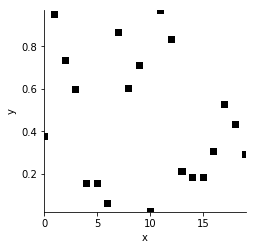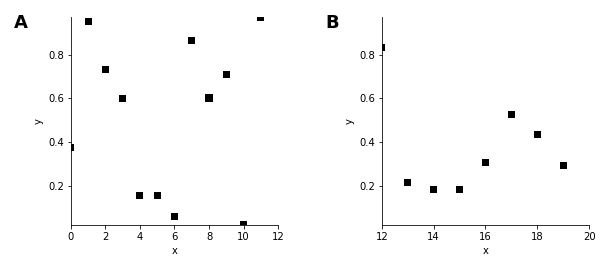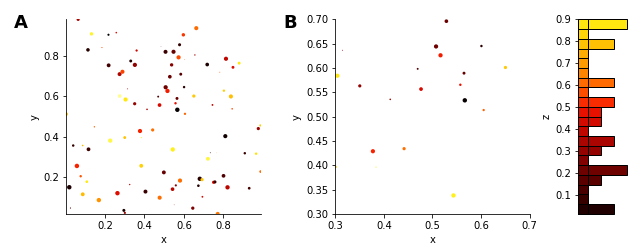Scatter¶

Title
Scatter Element
Dependencies
Matplotlib
Backends
Matplotlib
Bokeh
In :
import numpy as np
import holoviews as hv
from holoviews import dim

hv.extension('matplotlib')The Scatter element visualizes as markers placed in a space of one independent variable, traditionally denoted as x, against a dependent variable, traditionally denoted as y. In HoloViews, the name 'x' is the default dimension name used in the key dimensions (kdims) and 'y' is the default dimension name used in the value dimensions (vdims). We can see this from the default axis labels when visualizing a simple Scatter element:

In :
np.random.seed(42)
coords = [(i, np.random.random()) for i in range(20)]
scatter = hv.Scatter(coords)

scatter.opts(color='k', marker='s', s=50)
Out:Here the random y values are considered to be the 'data' whereas the x positions express where those values are located (compare this to how Points elements are defined). In this sense, Scatter can be thought of as a Curve without any lines connecting the samples and you can use slicing to view the y values corresponding to a chosen x range:

In :
scatter[0:12] + scatter[12:20]
Out:A Scatter element must always have at least one value dimension but that doesn't mean additional value dimensions aren't supported. Here is an example with two additional quantities for each point, declared as the vdims 'z' and 'size' visualized as the color and size of the dots, respectively:

In :
np.random.seed(10)
data = np.random.rand(100,4)

scatter = hv.Scatter(data, vdims=['y', 'z', 'size'])
scatter = scatter.opts(color='z', s=dim('size')*20)
scatter + scatter[0.3:0.7, 0.3:0.7].hist('z')
Out:In the right subplot, the hist method is used to show the distribution of samples along our first value dimension, (y).

The marker shape specified above can be any supported by matplotlib, e.g. s, d, or o; the other options select the color and size of the marker.

Note: Although the Scatter element is superficially similar to the Points element (they can generate plots that look identical), the two element types are semantically quite different: Points are used to visualize data where the y variable is dependent. This semantic difference also explains why the histogram generated by hist call above visualizes the distribution of a different dimension than it does for Points.

This difference means that Scatter naturally combine elements that express dependent variables in two-dimensional space such as the Chart types, such as Curve. Similarly, Points express a independent relationship in two-dimensions and combine naturally with Raster types such as Image.

For full documentation and the available style and plot options, use hv.help(hv.Scatter).